## *DEFORMATION PLASTICITY

Keyword type: model definition, material

This option defines the elasto-plastic behavior of a material by means of the generalized Ramberg-Osgood law. The one-dimensional model takes the form: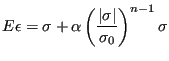(620)

where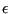is the logarithmic strain and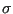the Cauchy stress. In the present implementation, the Eulerian strain is used, which is very similar to the logarithmic strain (about 1.3 % difference dat 20 % engineering strain). All coefficients may be temperature dependent.

First line:

• *DEFORMATION PLASTICITY

Following line:

• Young's modulus (E).
• Poisson's ratio (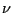).
• Yield stress (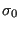)
• Exponent (n).
• Yield offset (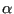).
• Temperature.
Repeat this line if needed to define complete temperature dependence.

Example:

*DEFORMATION PLASTICITY
210000.,.3,800.,12.,0.4


defines a Ramberg-Osgood law. No temperature dependence is introduced.

Example files: beampl.